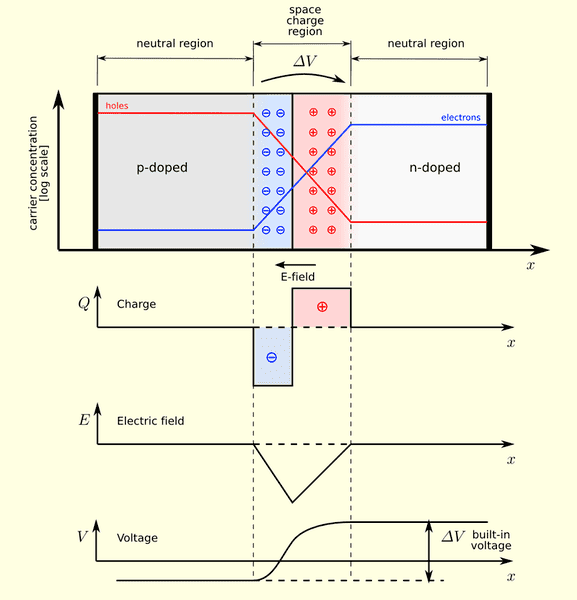# Questions about the Potential and E-Field directions of P-N junction

SandboHi, on re-visiting some basic ideas about PN junctions, I found I am confused with the depletion region diagram:

I understand that the depletion region originated from the diffusion current at the local contact of the p and n type material, the electrons diffuse from the n type to p type building a local E-Field, the field intensity is the strongest at the contact interface and decreases gradually towards the end of the material of both p and n type materials.

1. From the diagram above, why is the potential of the material remains a negative(towards the end of p-type) and positive(towards the n-type)?
Isn't it only the depletion region that will encounter a change in potential?
How come the whole right and whole left are positive and negative after all?2. As the +ve ions are left at the right(n side) and -ve ions are created on the left(p side), I suppose the E-field points from the right to left(n to p), the arrow indicates this and makes sense.
However, from the E-Field graph, the intensity is a negative value, does it mean they are actually taking "right" as positive?

Thanks for reading## Answers and Replies

Homework Helper
Gold Member
1. E points to the -x direction so E(x) is negative.

2. Since the electric field is the (negative) derivative of V wrt x, then V integrates to a constant once E = 0.